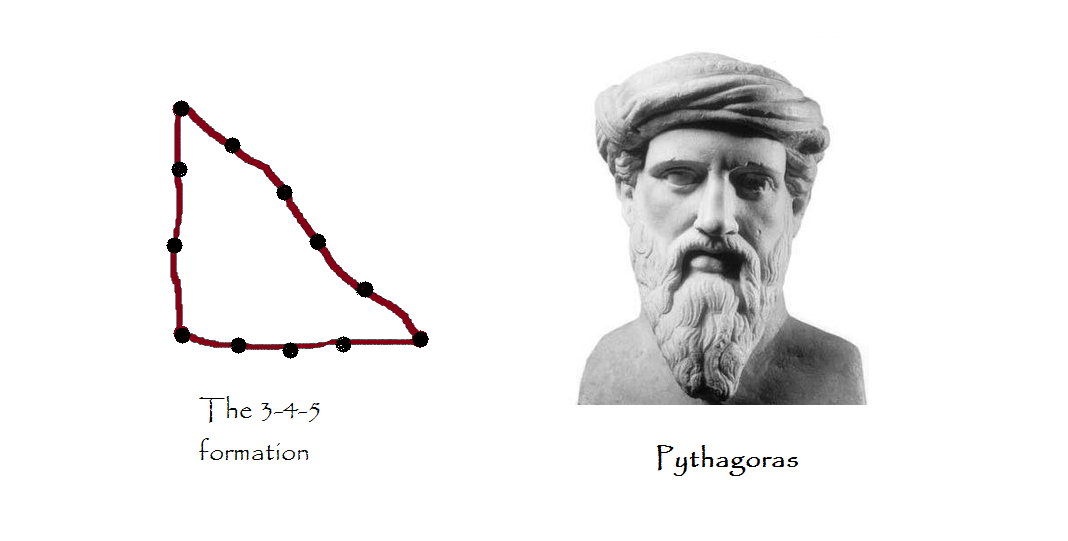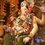# How did Pythagoras Arrive at his Famous Theorem?Arguably, one of the most important theorems ever in mathematics is the Pythagorean Theorem, also known as the Pythagoras' Theorem. Pythagoras was a Greek mathematician genius who believed that Number rules the universe & he gave numerical values to many objects & ideas.

He traveled extensively during his youth including Egypt. There he saw Rope Stretchers at work. These were the engineers who helped build the pyramids. Pythagoras observed the ropes they carried, which contained $12$ evenly spaced knots. When they stood in a $3-4-5$ formation, a right angle would emerge instantaneously enabling them to lay their foundations accurately.

Pythagoras began experimenting with these technique on a smaller scale by making drawings on the ground. Once, he found that the sum of the areas of the smaller two squares equaled the area of the area of the larger square. He further experimented on this discovery & arrived at his famed theorem.Note by Ameya Salankar
7 years, 3 months ago

This discussion board is a place to discuss our Daily Challenges and the math and science related to those challenges. Explanations are more than just a solution — they should explain the steps and thinking strategies that you used to obtain the solution. Comments should further the discussion of math and science.

When posting on Brilliant:

• Use the emojis to react to an explanation, whether you're congratulating a job well done , or just really confused .
• Ask specific questions about the challenge or the steps in somebody's explanation. Well-posed questions can add a lot to the discussion, but posting "I don't understand!" doesn't help anyone.
• Try to contribute something new to the discussion, whether it is an extension, generalization or other idea related to the challenge.

MarkdownAppears as
*italics* or _italics_ italics
**bold** or __bold__ bold
- bulleted- list
• bulleted
• list
1. numbered2. list
1. numbered
2. list
Note: you must add a full line of space before and after lists for them to show up correctly
paragraph 1paragraph 2

paragraph 1

paragraph 2

[example link](https://brilliant.org)example link
> This is a quote
This is a quote
    # I indented these lines
# 4 spaces, and now they show
# up as a code block.

print "hello world"
# I indented these lines
# 4 spaces, and now they show
# up as a code block.

print "hello world"
MathAppears as
Remember to wrap math in $$ ... $$ or $ ... $ to ensure proper formatting.
2 \times 3 $2 \times 3$
2^{34} $2^{34}$
a_{i-1} $a_{i-1}$
\frac{2}{3} $\frac{2}{3}$
\sqrt{2} $\sqrt{2}$
\sum_{i=1}^3 $\sum_{i=1}^3$
\sin \theta $\sin \theta$
\boxed{123} $\boxed{123}$